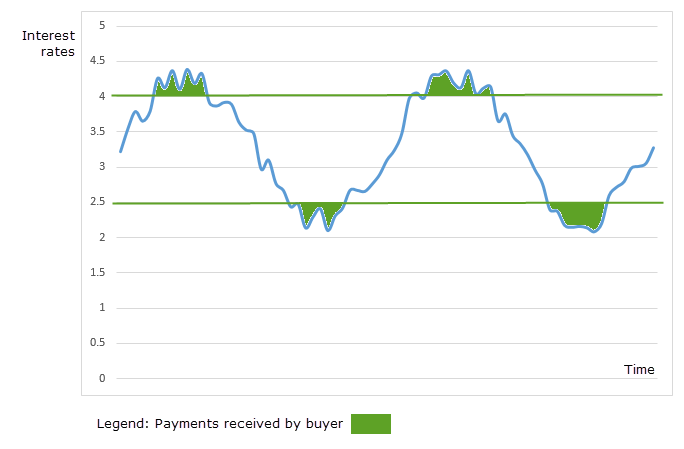# Interest rate collar

An interest rate collar is a combination of an interest rate cap and an interest rate floor.

## A risk averse manager

Suppose that a fund manager is concerned about interest rate volatility, that is, both increases and decreases in the rate beyond some specified value. To reduce the impact of interest rate movements (volatility), the manager has bought an interest rate cap - set at 4 percent, and bought an interest rate floor set at 2.5 percent as illustrated in figure 1.Fig 1: Interest rate collar - a risk averse manager has bought a collar comprising the purchase of a interest rate cap at 4% and the purchase of an interest rate floor at 2.5%.

If, during the time period of the collar, interest rates exceed the 4 per cent cap rate, and/ or fall below the 2.5 percent floor rate, then the manager will receive payments as shown by the green areas in figure 1. If interest rates stay within the 2.5 percent to 4.0 percent range, then no cash is received by the buyer. In addition, the buyer has paid a premium for the cap and the collar and this cost must be factored into the overall payoff of the strategy.

## Simulating the interest rate curve

The curve in figure 1 is a mixture of a sine wave and random drawings from a uniform $U(0,1)$ distribution. After some experimentation, the mean was moved by +3, and a scaled $U(0,1)$ vector added (scaling 0.5).

Starting with an x vector of degrees in the range 0 to 720, the Excel formula is

• =SIN(RADIANS(x))+(0.5*RAND())+3

• Published: 23 September 2014
• Revised: Tuesday 25th of February 2020 - 09:20 AM, [Australian Eastern Standard Time (EST)]